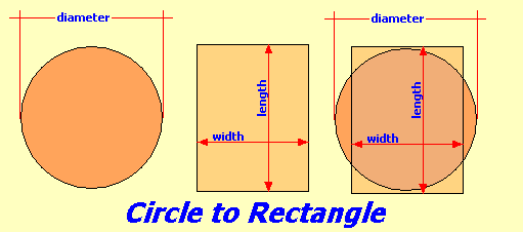Spike's Calculators

# Changing a Circle to a Rectangle

Convert the area of a circle into an rectangle shaped area of the same size. Works much the same way as a circle to square conversion but you will have to enter the width of the rectangle in addition to the circle's diameter.### Circle Formula's

`R = D ÷ 2`
where R = radius, D = diameter

Area;
`A = π * D² ÷ 4`
where A = area, π = 3.14159..., D = diameter

Circumference;
`C = 2 * π * D ÷ 2`
where C = circumference, π = 3.14159...., D = diameter

### Rectangle Formula's

Area
`A = length * width`

Length
`L = A ÷ W`
where L= length, A = area, W = width

Perimeter
`P = length * 2 + width * 2`

### Circle to a Rectangle

Select unit:
diameter of the circle {{selectedunit.m1}}
width of rectangle {{selectedunit.m1}}
decimal rounding # of digits in results

#### Results:

 circle's radius {{selectedunit.m1}} area of the circle {{selectedunit.m1}}² circumference of the circle {{selectedunit.m1}} length of rectangle {{selectedunit.m1}} perimeter of the rectangle {{selectedunit.m1}}

#### Calculation

1. enter the diameter of a circle
2. the width of the rectangle
3. number of decimal places required in results

#### Results

2. the area of the circle
3. the perimeter of the circle (circumference)
4. the length of the rectangle
5. perimeter of the rectangle